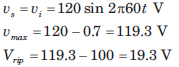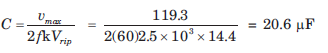Courses

# Diode Circuits - MCQ Test

## 15 Questions MCQ Test Mock Tests Electronics & Communication Engineering GATE 2020 | Diode Circuits - MCQ Test

Description
This mock test of Diode Circuits - MCQ Test for Railways helps you for every Railways entrance exam. This contains 15 Multiple Choice Questions for Railways Diode Circuits - MCQ Test (mcq) to study with solutions a complete question bank. The solved questions answers in this Diode Circuits - MCQ Test quiz give you a good mix of easy questions and tough questions. Railways students definitely take this Diode Circuits - MCQ Test exercise for a better result in the exam. You can find other Diode Circuits - MCQ Test extra questions, long questions & short questions for Railways on EduRev as well by searching above.
QUESTION: 1

### In the question a circuit and a waveform for the input voltage is given. The diode in circuit has cutin voltage Vγ = 0. Choose the option for the waveform of output voltage vo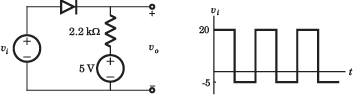​ ​ ​

Solution:

Diode is off for vi  < 5 V. Hence vo = 5 V.

For vi > 5 V, vo = vi .Therefore (D) is correct option.

QUESTION: 2

### In the question a circuit and a waveform for the input voltage is given. The diode in circuit has cutin voltage Vγ = 0. Choose the option for the waveform of output voltage vo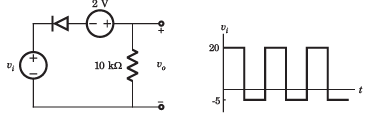Solution:

Diode will be off if vi + 2 > 0.Thus vo = 0

For v+ 2  < 0 V, vi  < -2, vo = vi + 2 = -3V

Thus (C) is correct option

QUESTION: 3

### In the question a circuit and a waveform for the input voltage is given. The diode in circuit has cutin voltage Vγ = 0. Choose the option for the waveform of output voltage vo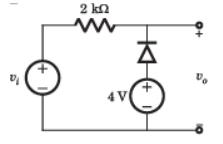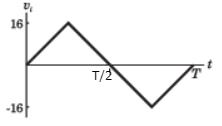Solution:

For vi  < 4 V the diode is ON and output vo = 4 V.

For vi  >4 V diode is off and output vo = vi.
Thus (D) is correct option.

QUESTION: 4

In the question a circuit and a waveform for the input voltage is given. The diode in circuit has cutin voltage Vγ = 0. Choose the option for the waveform of output voltage vo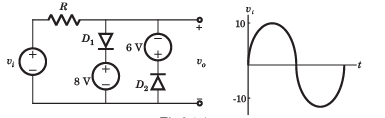Solution:

During positive cycle when vs < 8V both diode are OFF vo = vs . For vs > 8V , vo = 8V , D1 is ON.During
negative cycle when |vs| < 6 V, both diode are OFF, vo = vs . For |vs| > 6V , D2 is on v0 = -6V .Therefore (C) is
correct.

QUESTION: 5

For the circuit in fig. P.3.1.5, let cutin voltage Vγ = 0.7 V. The plot of vo verses vi for -10 ≤ vi ≤10 V is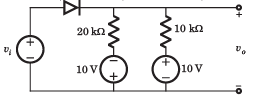Solution: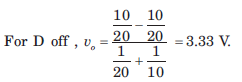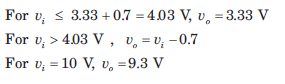QUESTION: 6

The circuit inside the box in fig. contains only resistor and diodes. The terminal voltage vo is connected to some point in the circuit inside the box. The largest and smallest possible value of vo most nearly to is respectively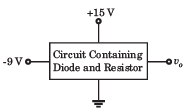Solution:

The output voltage cannot exceed the positive power supply voltage and cannot be lower than the negative power supply voltage.

QUESTION: 7

In the voltage regulator circuit in fig. the maximum load current iL that can be drawn is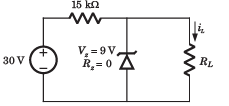Solution:

At regulated power supply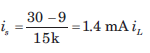will remain less than 1.4 mA.

QUESTION: 8

In the voltage regulator shown in fig. the power dissipation in the Zener diode is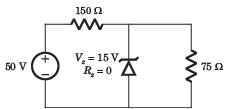Solution: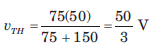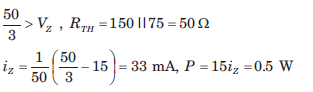QUESTION: 9

The Q-point for the Zener diode in fig. is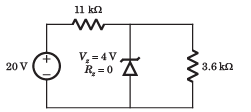Solution: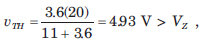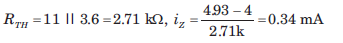QUESTION: 10

In the voltage regulator circuit in fig  the power rating of Zener diode is 400 mW. The value of RL that will establish maximum power in Zener diode is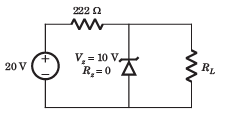Solution: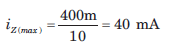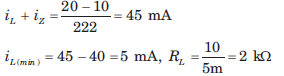QUESTION: 11

In the voltage regulator circuit in fig.  the Zener diode current is to be limited to the range 5 ≤ iz ≤100 mA.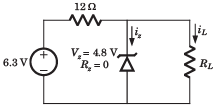Que: The range of possible load current is

Solution:

Current through 12 Ω resistor is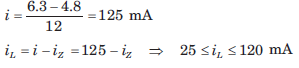QUESTION: 12

In the voltage regulator circuit in fig.  the Zener diode current is to be limited to the range 5 ≤ iz ≤100 mA.Que: The range of possible load resistance is

Solution: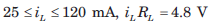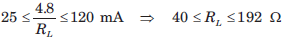QUESTION: 13

In the voltage regulator circuit in fig.  the Zener diode current is to be limited to the range 5 ≤ iz ≤100 mA.: The power rating required for the load resistor is

Solution: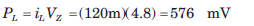QUESTION: 14

The secondary transformer voltage of the rectifier circuit shown in fig. is vs = 60 sin 2π 60tV. Each diode has a cut in voltage of Vγ = 0.6 V. The ripple voltage is to be no more than Vrip = 2 V. The value of filter capacitor will be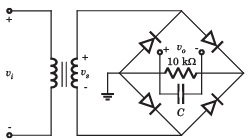Solution:

vs = 60 sin 2π 60tV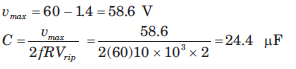QUESTION: 15

The input to full-wave rectifier in fig. is vi = 120 sin2π60t V. The diode cutin voltage is 0.7 V. If the output voltage cannot drop below 100 V, the required value of the capacitor is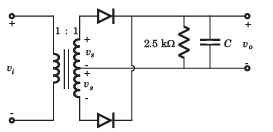Solution:

Full wave rectifier